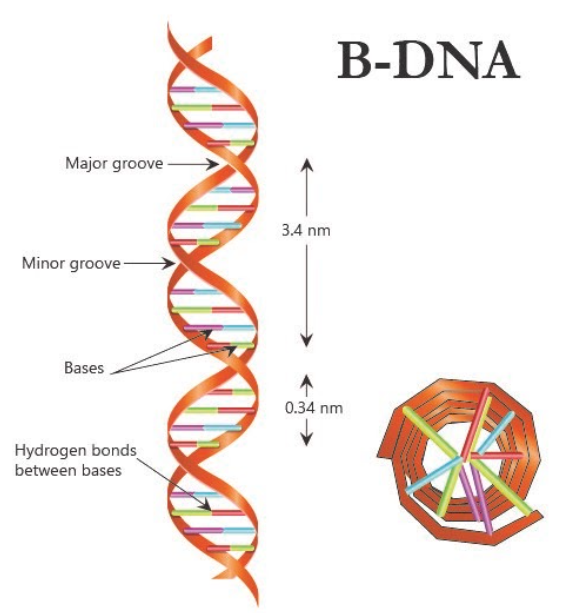If the length of E.coli DNA is 1.36 mm, can you calculate the number of base pairs in E.coli?Verified
119.1k+ views
Hint: DNA is a polymer that is made up of the repeating unit nucleotides. These nucleotides contain nitrogenous bases that pair with each other and are known as base pairs (bps). The DNA of Escherichia coli is double-stranded circular DNA.

The length of DNA is usually represented in terms of the number of nucleotides (or a pair of nucleotides referred to as base pairs) present in it. The structure of DNA is such that between two consecutive base pairs, a distance of 0.34 nm (0.34×${ 10 }^{ -9 }$ m) is present. Thus, one can find the total length of a DNA of an organism by multiplying the total number of base pairs with a distance between two consecutive base pairs. Since we are given the length of the DNA of E.coli as 1.36 mm, we can divide this value by the distance between two consecutive base pairs to get the total number of base pairs present.
Total number of base pairs= 1.36mm/0.34x${ 10 }^{ -6 }$mm
Total number of base pairs= 4x${ 10 }^{ 6 }$ base pairs
-The number of base pairs in Bacteriophage lambda is 48502 base pairs, in Bacteriophage $\phi$ ×174 is 5386 base pairs, and in a haploid cell of a human is 3.3 x ${ 10 }^{ 9 }$ base pairs.
-The length of DNA in a diploid human cell is calculated as 6.6 × ${ 10 }^{ 9 }$ bp × 0.34 × ${ 10 }^{ -9 }$m/bp, and it comes out to be approximately 2.2 meters.